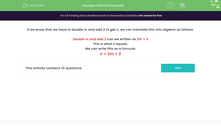# Find the Correct Formula

In this worksheet, students will select the correct formula that matches the given rule.Key stage:  KS 3

Curriculum topic:   Algebra

Curriculum subtopic:   Use Standard Mathematical Formulae

Popular topics:   Algebra worksheets, Year 7 Algebra worksheets

Difficulty level:#### Worksheet Overview

A formula tells you how to work out something given another piece of information.

The formula F = 1.8C + 32 is used to convert a temperature in Centigrade to one in Fahrenheit.

When C = 10, we substitute this value into the formula to get:

F = 1.8 x 10 + 32

F = 18 + 32

F = 50

This means that 10º C is the same as 50º FThe plural of formula can be written as either formulas or formulae.

You will come to know many different formulae in both maths and science work.

Here's another example of a formula:

If we know that we have to double m and add 3 to get n, we can translate this into algebra as follows:

Double m and add 3 can we written as 2m + 3

This is what n equals.

We can write this as a formula:

n = 2m + 3

Let's have a go at some questions now.

### What is EdPlace?

We're your National Curriculum aligned online education content provider helping each child succeed in English, maths and science from year 1 to GCSE. With an EdPlace account you’ll be able to track and measure progress, helping each child achieve their best. We build confidence and attainment by personalising each child’s learning at a level that suits them.

Get started#### Popular Maths topics

••••